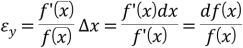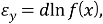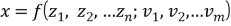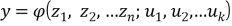Mixture formation plays
19iewhere the sign d after differentiation should be replaced by Δ.

## 1.5 Methods of the theory of correlation

Apply when the two values x and y are not linked func–tional dependence, but are probabilistic relationship. This relationship is ap–lyaetsya usually when there is general random factors affecting as one and to another value, along with other unequal values for both random factors, such as,;.

With such a relationship between x and y makes sense to talk about the law of distribution of these variables on the probability with which there are certain combinations of values. To do this, consider the function that creates the expectation of a size at different fixed values of the other. These functions are called regression, and their graphic representation in the plane (x, y) (regression line) is the locus of the centers of conditional distributions (center of mass), corresponding to a given value of one variable.

Along the lines of the regression line review and conditional variances, which is a measure of dispersion of a value at fixed values of the other.

By analyzing these relationships allows to solve the problem of sound prediction, ie to find the extent to which reliability with preset contains the value we are interested if other related quantities are of some importance.